Courses

# Differential Equations - 18

## 20 Questions MCQ Test Topic-wise Tests & Solved Examples for IIT JAM Mathematics | Differential Equations - 18

Description
This mock test of Differential Equations - 18 for Mathematics helps you for every Mathematics entrance exam. This contains 20 Multiple Choice Questions for Mathematics Differential Equations - 18 (mcq) to study with solutions a complete question bank. The solved questions answers in this Differential Equations - 18 quiz give you a good mix of easy questions and tough questions. Mathematics students definitely take this Differential Equations - 18 exercise for a better result in the exam. You can find other Differential Equations - 18 extra questions, long questions & short questions for Mathematics on EduRev as well by searching above.
QUESTION: 1

### If f1 and f2 be the integrating factors of the differential equations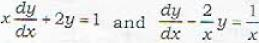respectively,

Solution:

The given differential equations are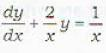......(i)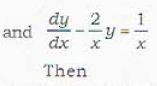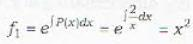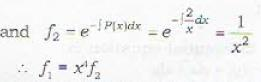QUESTION: 2

### Which of the following statements is correct?

Solution:

The infinite number of differential equations given by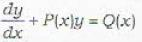for infinitely many different choices of Q(x), possess the same integrating factor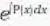QUESTION: 3

### The integrating factor of the differential equation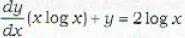is given by

Solution:

The given ciifferential equation is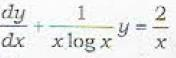The required Integrating factor is given by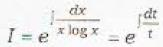[log x = t]
= e log t  = t = log x

QUESTION: 4

The integrating factor of the differential equation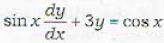is calculated as

Solution:

The given differential equation is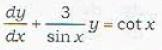The required Integrating factor is given by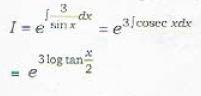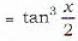QUESTION: 5

The differential equation of the type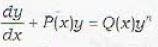is known a

Solution:
QUESTION: 6

Which of the following transformations reduces the differential equation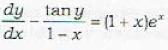sec y into the form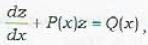by the substitution

Solution:

The given differential equation can be rewritten as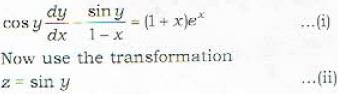This implies that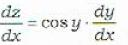∴ differential equation (i) reduces to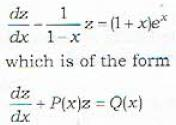Remark : Observe that the transformation (ii) is suggested by form (i) of given differential eouation.

QUESTION: 7

Which of the following transformations reduces the differential equation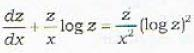into the form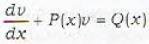by the substitution

Solution:

Rewrite the given differential equation as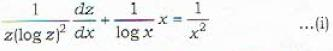This suggests that the required transformation is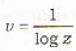QUESTION: 8

The transformation v = 1/y transforms which the following differential equations into the form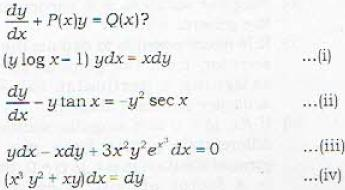Solution:
QUESTION: 9

The general solution of the differential equation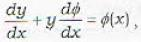where φ is a function of x alone, is given by

Solution:

The given differential equation is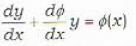.....(i)
Its Integrating Factor is given by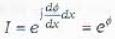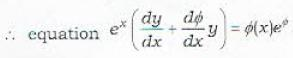becomes exact.
This implies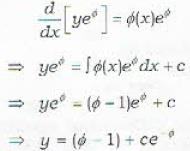QUESTION: 10

The equation xdy = ydx represents the family of

Solution:

The differential equation is x dy = y dx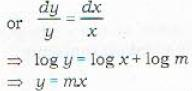This represents the family of straight lines passing through the origin.

QUESTION: 11

The equation xdy + ydx = 0 represents a family of

Solution:

The given differential equation can be written as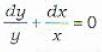⇒ log y + log x = log c
⇒ yx = c
This represents the family of hyperbolas.

QUESTION: 12

The one parameter family y = or can be written as

Solution:

The given one parameter family is
y = cx2     .....(i)
Differentiating (i) w.r. t. x, we get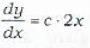....(ii)
On eliminating (c) from (i) and (ii). we get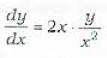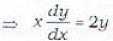QUESTION: 13

A curve is called an isogonal trajectoiy or an x trajectory of family f(x, y, c) = 0 if

Solution:

Statement (c) gives the correct definition of an isogonal trajectory.

QUESTION: 14

In order to find a family of orthogonal trajectories of the family of curves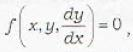which of the following replacements is made

Solution:

Given family of the curves is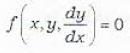.....(i)
Its slope is dy/dx. If m is the slope of the orihogonal trajectories of the family (i) then we should have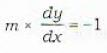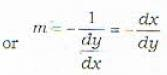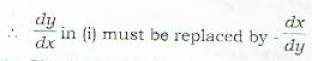QUESTION: 15

The orthogonal trajectories of the given family of curves y = cx2 are given by

Solution:

Then given family of curves is
y = cxk  ....(i)
.v —
Let us first find the differential equation satisfied by the family (i). For this we differentiate (i) w.r. t.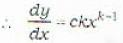∴ The differential equation of the orthogonal trajectories will be obtained on replacing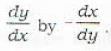⇒ Orthogonal trajectories are given by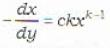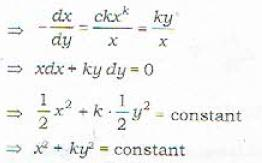QUESTION: 16

Which one of the following is a general solution of the differential equation xp2 + (y - x)p - y = 0 where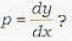Solution:

The given differential equation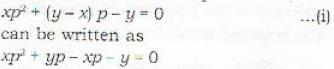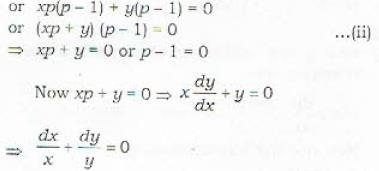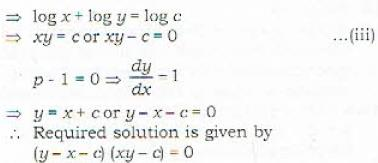QUESTION: 17

Which one of the following is a general solution of the differential equation p2 - 2p cos hx + 1 = 0?

Solution:

Hint: Write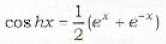and do as in the previous question.

QUESTION: 18

Which one of the following is a general solution of the differential equation y = 2px + p2y ?

Solution:

1. If the given equation in x, y and p can be solved for y, then we shall have
y = f(x,p)    ...(i)
Differentiate (i) w.r. t. x. This will lead to a differentia! equation in p and x. Solve it for p and then eliminate p from the resulting equation and (i).
2. If the given equation in x, y and p can be solved for x, then we shail have
x = f(y, p) .......(ii)
Differentiate (ii), w.r. t. y and follow the same procedure as in case - i.
Now solution of the problem 82.
The given differential equation is
y = 2 px + p2y   ...... (iii)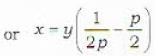... (iv)
which is solved for x, i.e. it expresses x as a function of y and p.
Now differentiating (iv) w.r. t. y, we get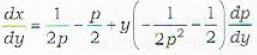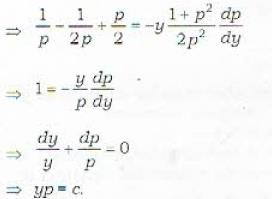Now eliminating p from equations (iii) and (v), we get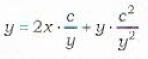or y2 = 2cx + c2

QUESTION: 19

Which one of the following is a general solution of the differential equation y - 2px = f(xp2)?

Solution:

The given differential equation is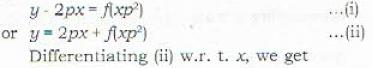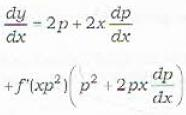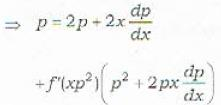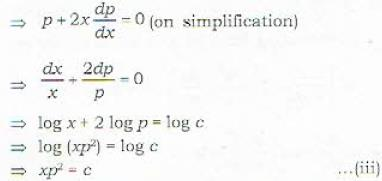Now eliminating p from equations (i) and (iii).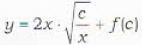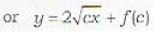QUESTION: 20

The differential equation of the form y = x F(p) + f(p) is known as

Solution: